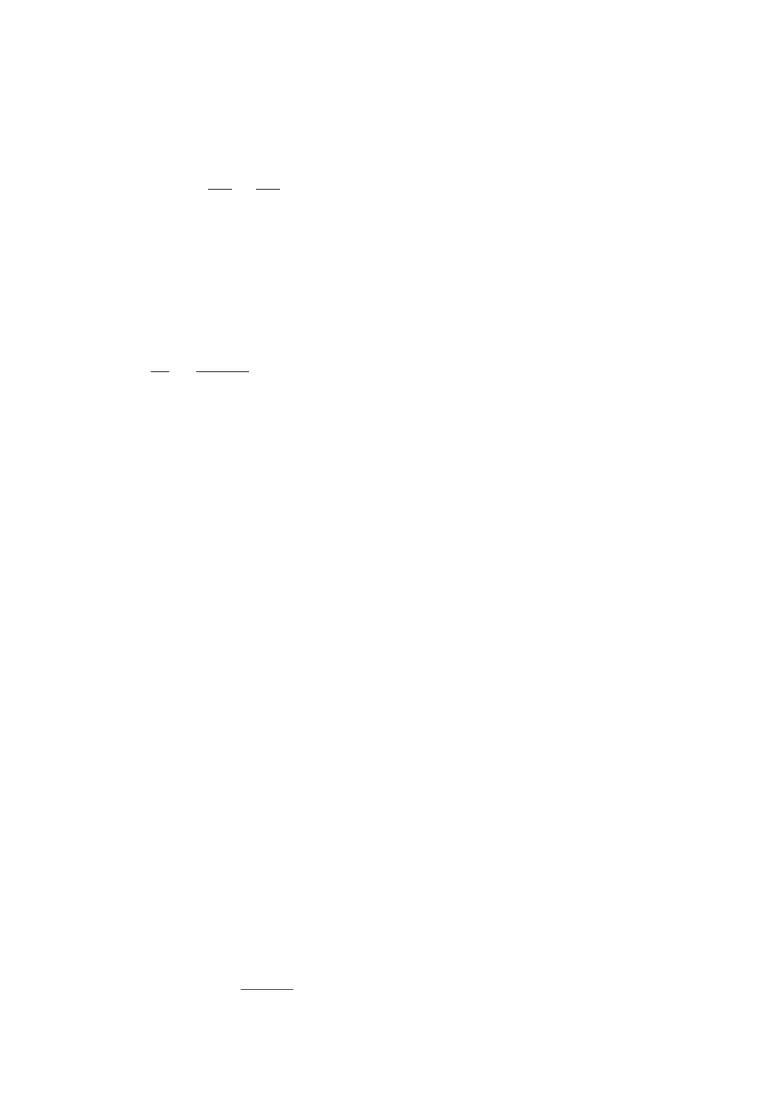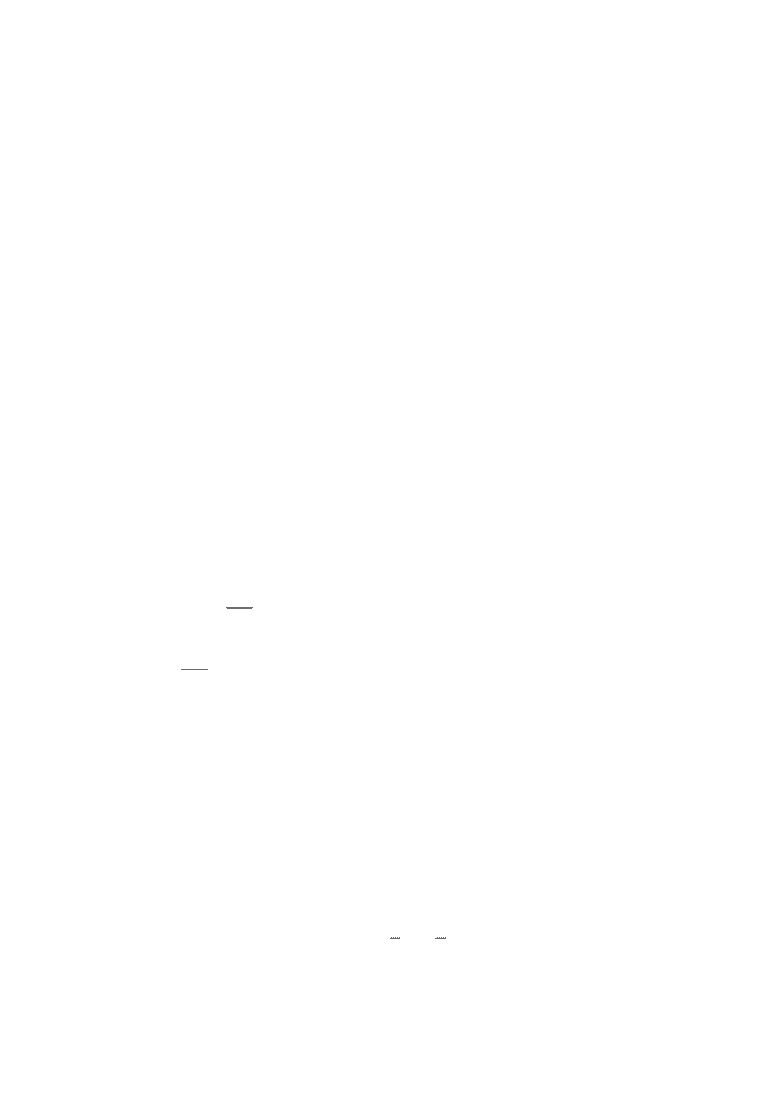Study Guides (390,000)
CA (150,000)
UTSC (10,000)
Mathematics (1,000)
MATA32H3 (100)
All (60)
Final

# MATA32H3 Final: MATA32H3 Final Exam 2012 Fall SolutionsExam

Department
Mathematics
Course Code
MATA32H3
Professor
All
Study Guide
Final

This preview shows pages 1-2. to view the full 7 pages of the document.1.
According to the given information
For
11
0
0
32
3|
ln3 ln3
x
xdx = =
Therefore C is correct.
2.
According to the given information
For
22
4*2
( 3)
du t
dt t
= −
Therefore D is correct.
3.
According to the given information
For
5
1000*1.02 1104=
,then
Therefore B is correct.
4.
For
33
2 22
00
4 (6 2 )(6 ) 4 (6 ) (6 )t ttdt ttdtt+ += + +
∫∫
Therefore E is correct.
5.
For
32
' 64 120yxx=−+
and
2
" 192 240y xx=−+
then
Let y”>0
Therefore D is correct.
6.
For
2
2
2
'
2 *' 6
y xy
y xy y y
++ =
and x=4 , y=2

Only pages 1-2 are available for preview. Some parts have been intentionally blurred.Therefore C is correct.
7.
For
() 2ln()fx xxx= +
and f’(x)=2ln(x)+2+1
Therefore C is correct.
8.
Let (p*q)’(q)=0
Therefore B is correct.
9.
For
5
1.04(1 ) 1.24x+=
Therefore B is correct.
10.
For
,so
Let
405
5qq
=
Therefore D is correct.
11.
For (ii),(iii) is correct
Therefore C is correct.
1.(a)
for
2 2 23
14
( 4 4) 4 4+
23
xx x xx
dx e e x x dx e x xe e C= + + = ++
∫∫
x2
( e +2x)